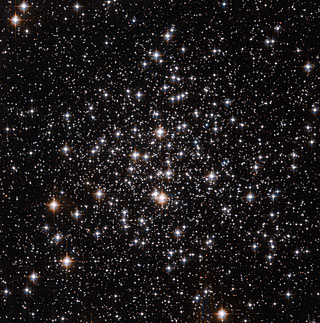# Going Virial

In Stars by Brian Koberlein0 Comments

There’s an interesting theorem in physics known as the virial theorem.  One version of this theorem states that if you have hundreds or thousands of stars clustered together, then the average kinetic energy of a particular star is about equal to half the average gravitational potential energy of a star.  What this means is that given a cluster of stars (such as the globlular cluster M71 pictured below) the typical speed of a star is proportional to the total mass of the cluster.  As a result the virial theorem is a way we can determine the mass of star clusters.

There are a couple of catches, however.  The first is that we can only really measure a star’s radial motion toward or away from us using the Doppler effect.  The second is that we can really only get the radial velocities of the bright stars in the clusters.  This means we have to make a few assumptions if we want to use the virial theorem.

The first assumption is that the cluster is stable.  As long as the cluster isn’t in the process of forming, or otherwise behaving strangely, this is a valid assumption.  The second is to assume all the stars have the same mass.  This isn’t really true, but it usually is a decent approximation.  The third is to assume the stars for which you measure velocity have typical motions.  If you happened to choose particularly fast or slow stars that would skew your answer.

If these assumptions are valid, then we can measure the radial speeds of a dozen stars and get a reasonable measure of the cluster’s mass.  In the case of M71, that turns out to be about 17,000 solar masses.